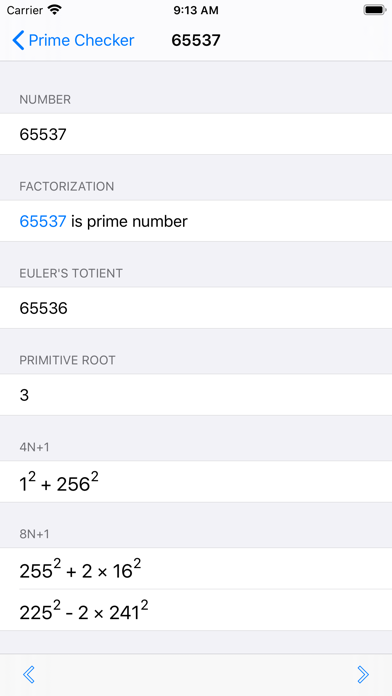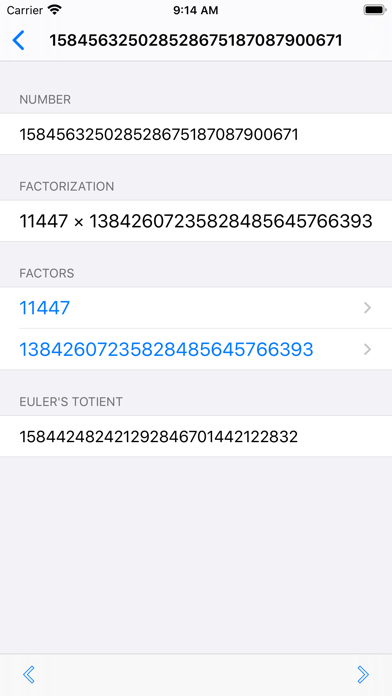## Prime Checker - Lumos Educational App Store51
Price -Free
\$0

#### DESCRIPTION:

This application calculate values of given number which are described below: Integer factorization Euler's totient function Primitive root (if exist) x, y where x^2 + y^2 = p if p is 4n+1 x, y where x^2 + 3y^2 = p if p is 3n+1 x, y where x^2 + 2y^2 = p if p is 8n+1 or 8n+3 x, y where x^2 - 2y^2 = p if p is 8n+1 or 8n+7 This application uses Fermat algorithm, Pollard's rho algorithm and Lenstra's ECM for factorization, Miller–Rabin algorithm for primality test

#### OVERVIEW:

Prime Checker is a free educational mobile app By Heart Solutions, Inc..It helps students practice the following standards .

This page not only allows students and teachers download Prime Checker but also find engaging Sample Questions, Videos, Pins, Worksheets, Books related to the following topics.

Developer: Heart Solutions, Inc.

Developer URL: NA

Software Version: 3.1

Category: Education

Release Date: 2010-08-30T21:23:16Z#### Are you the Developer?EdSearch WebSearch